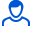Content: teorver_5562.doc (28.00 KB)

Positive responses: 0
Negative responses: 0

Refunds: 05562. x̅=5, n=25, σ=8. Determine the confidence interval for estimating with reliability γ=0.95 the unknown mathematical expectation a of the normally distributed feature X of the general population, if the sample mean x̅в=5, the sample size n=25 and the general standard deviation σ=8 are known.
Detailed solution. Decorated in Microsoft Word 2003 (Quest decided to use the formula editor)
No feedback yet